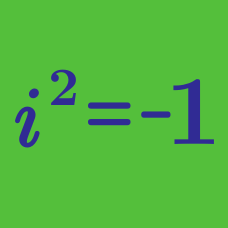Algebra

# Complex Numbers Arithmetic

What is the absolute value of the complex number $12+5i?$

Details and assumptions

$i$ is the imaginary number that satisfies $i^2 = -1$.

Is it true that $\Re\big(\Im(z)\big) = \Im\big(\Re(z)\big)$ for all complex numbers $z?$

What is the value of $\left(9 + \sqrt{-81}\right)\left(20 - \sqrt{-400}\right)?$

Simplify $\frac{4+2i}{2-i}$.

Details and assumptions
$i$ is the imaginary number that satisfies $i^2 = -1$.

Which set of complex numbers satisfy the equation $iz + \overline{z} = 0$?

Hint: Start by letting $z = x + iy$.

×